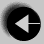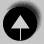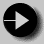## Recursive Functions

A recursive function is one which calls itself. This is another complicated idea which you are unlikely to meet frequently. We shall provide some examples to illustrate recursive functions.

Recursive functions are useful in evaluating certain types of mathematical function. You may also encounter certain dynamic data structures such as linked lists or binary trees. Recursion is a very useful way of creating and accessing these structures.

Here is a recursive version of the Fibonacci function. We saw a non recursive version of this earlier.

```
int fib(int num)
/* Fibonacci value of a number */
{       switch(num) {
case 0:
return(0);
break;
case 1:
return(1);
break;
default:  /* Including recursive calls */
return(fib(num - 1) + fib(num - 2));
break;
}
}```

We met another function earlier called power. Here is an alternative recursive version.

```
double power(double val, unsigned pow)
{
if(pow == 0)  /* pow(x, 0) returns 1 */
return(1.0);
else
return(power(val, pow - 1) * val);
}```

Notice that each of these definitions incorporate a test. Where an input value gives a trivial result, it is returned directly, otherwise the function calls itself, passing a changed version of the input values. Care must be taken to define functions which will not call themselves indefinitely, otherwise your program will never finish.

The definition of fib is interesting, because it calls itself twice when recursion is used. Consider the effect on program performance of such a function calculating the fibonacci function of a moderate size number.If such a function is to be called many times, it is likely to have an adverse effect on program performance.

Don't be frightened by the apparent complexity of recursion. Recursive functions are sometimes the simplest answer to a calculation. However there is always an alternative non-recursive solution available too. This will normally involve the use of a loop, and may lack the elegance of the recursive solution.Go to Arrays and PointersGo to IndexGo to Input and Output